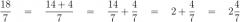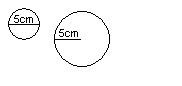favorited this question.
Since you said 'diameter', I assume you mean finding the area of a circle with diameter 5 meters. The formula for finding area of a circle is pi X (radius squared) OR pi X radius X radius. Diameter = 5m, Radius would be 5m/2 = 2.5m So the area of the circle would be 3.141592...Read more
favorited this question.
5cm radius or diameter? 5cm diameter (left), 5cm radius (right)favorited this question.
To convert a mixed number e.g. 4 8/9 to an improper fraction, 1. Take the whole number (4). 2. Multiply that by the denominator (9). (4 X 9 = 36) 3. Add this number to the numerator (8). (36 + 8 = 44) The improper fraction will be: 44/9 (44 is the number you got … Read more